# pyroomacoustics demo¶

In this IPython notebook, we demonstrate a few features of pyroomacoustics:

1. Its pythonic and convenient object-oriented interface.
2. The Room Impulse Response (RIR) generator.
3. Provided reference algorithms.

Below is a list of the examples (run all cells as some may depend on previous imports and results).

More information on the package can be found on the Github repo and from the paper, which can be cited as:

R. Scheibler, E. Bezzam, I. Dokmanić, Pyroomacoustics: A Python package for audio room simulations and array processing algorithms, Proc. IEEE ICASSP, Calgary, CA, 2018.

Let's begin by importing the necessary libraries all of which can be installed with pip, even pyroomacoustics!

In :
import numpy as np
import matplotlib.pyplot as plt
from scipy.io import wavfile
from scipy.signal import fftconvolve
import IPython
import pyroomacoustics as pra


## Creating a 2D/3D room¶

We can build an arbitrary room by specifing its corners on a plane.

In :
corners = np.array([[0,0], [0,3], [5,3], [5,1], [3,1], [3,0]]).T  # [x,y]
room = pra.Room.from_corners(corners)

fig, ax = room.plot()
ax.set_xlim([-1, 6])
ax.set_ylim([-1, 4]);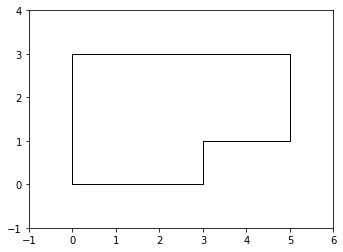If we wish to build a 3D room, we can "lift" the 2D shape by a specified height.

In :
room = pra.Room.from_corners(corners)
room.extrude(2.)

fig, ax = room.plot()
ax.set_xlim([0, 5])
ax.set_ylim([0, 3])
ax.set_zlim([0, 2]);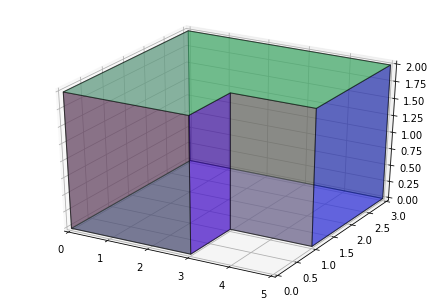We can conveniently add sources to the room using the add_source method. We can also set a numpy array as the source's signal.

The speech file comes from the CMU Arctic Database.

In :
# specify signal source

# add source to 2D room
room = pra.Room.from_corners(corners, fs=fs, ray_tracing=True, air_absorption=True)

fig, ax = room.plot()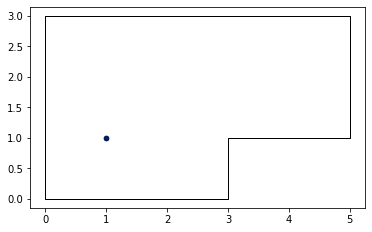And similarly add a microphone array.

In :
R = pra.circular_2D_array(center=[2.,2.], M=6, phi0=0, radius=0.1)

fig, ax = room.plot()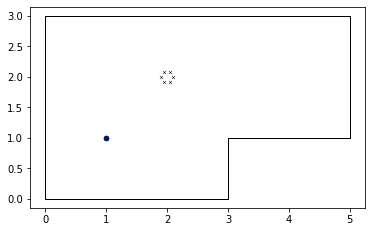## Room impulse response (RIR) generation and propagation simulation¶

Using the Image Source Model (ISM) we can compute the impulse response for each microphone. From the Room constructor it is possible to set the maximum ISM order and the absorption coefficient.

In :
# specify signal source

# set max_order to a low value for a quick (but less accurate) RIR
room = pra.Room.from_corners(corners, fs=fs, max_order=3, materials=pra.Material(0.2, 0.15), ray_tracing=True, air_absorption=True)
room.extrude(2., materials=pra.Material(0.2, 0.15))

# Set the ray tracing parameters

# add source and set the signal to WAV file content

R = np.array([[3.5, 3.6], [2., 2.], [0.5,  0.5]])  # [[x], [y], [z]]

# compute image sources
room.image_source_model()

# visualize 3D polyhedron room and image sources
fig, ax = room.plot(img_order=3)
fig.set_size_inches(18.5, 10.5)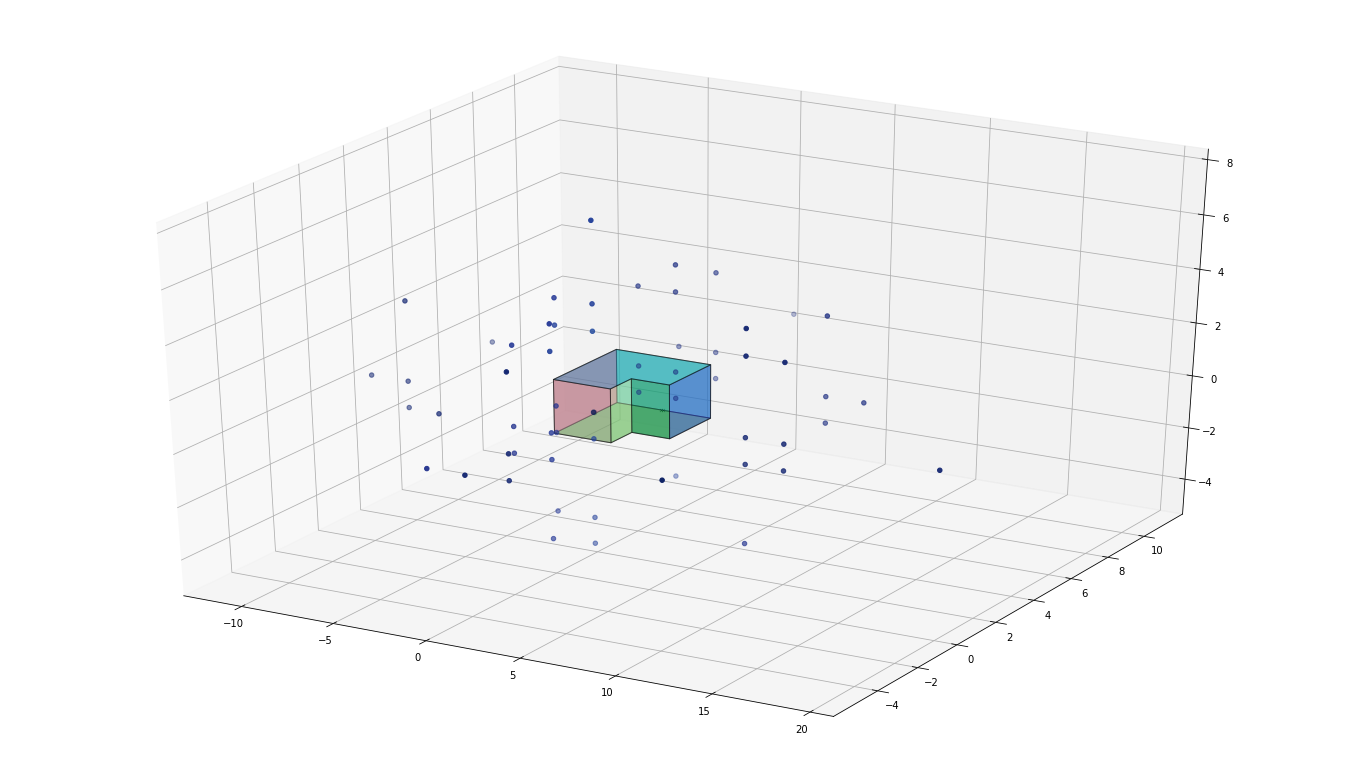Moreover, we can plot the RIR for each microphone once the image sources have been computed.

In :
room.plot_rir()
fig = plt.gcf()
fig.set_size_inches(20, 10)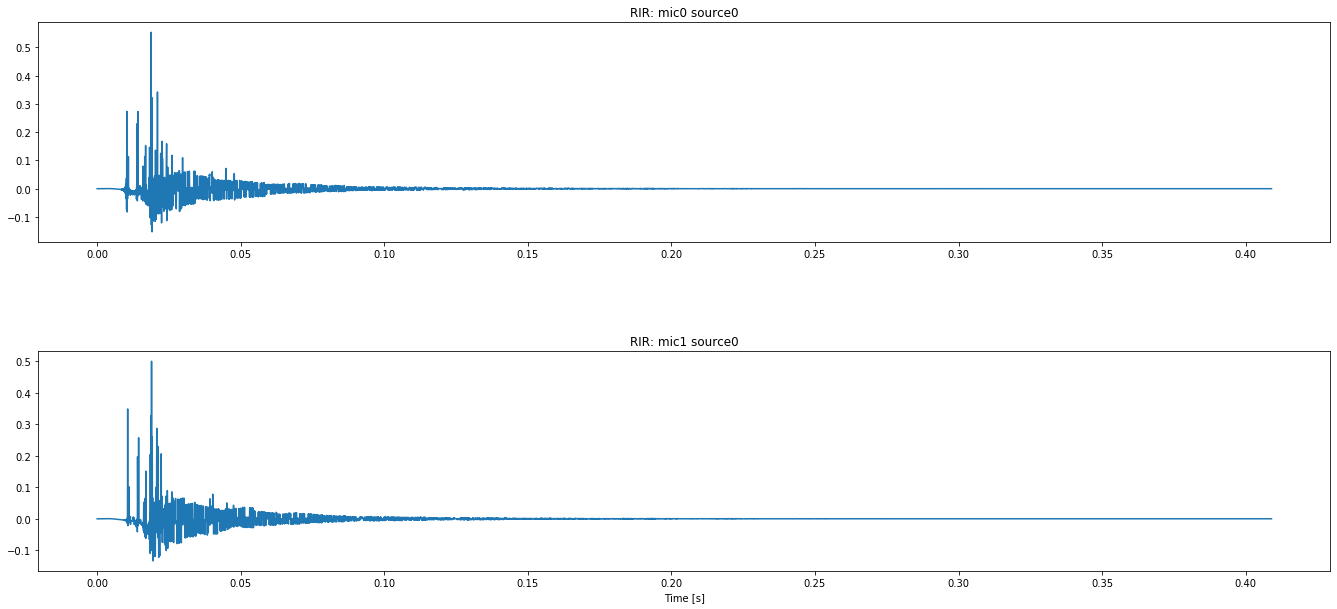We can now measure the reverberation time of the RIR.

In :
t60 = pra.experimental.measure_rt60(room.rir, fs=room.fs, plot=True)
print(f"The RT60 is {t60 * 1000:.0f} ms")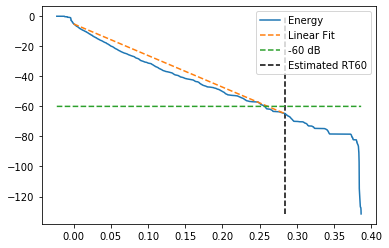The RT60 is 285 ms


Moreover, we can simulate our signal convolved with these impulse responses as such:

In :
room.simulate()
print(room.mic_array.signals.shape)

(2, 63584)


Let's listen to the output!

In :
# original signal
print("Original WAV:")
IPython.display.Audio(signal, rate=fs)

Original WAV:

Out:
In :
print("Simulated propagation to first mic:")
IPython.display.Audio(room.mic_array.signals[0,:], rate=fs)

Simulated propagation to first mic:

Out:

## Beamforming¶

Reference implementations of beamforming are also provided. Fixed weight beamforming is possible in both the time and frequency domain. Classic beamforming algorithms are included as special cases of the acoustic rake receivers, namely by including only the direct source we obtain DAS and MVDR beamformers.

The noise file comes from Google's Speech Command dataset.

In :
Lg_t = 0.100                # filter size in seconds
Lg = np.ceil(Lg_t*fs)       # in samples

# specify signal and noise source
fs, noise = wavfile.read("exercise_bike.wav")  # may spit out a warning when reading but it's alright!

# Create 4x6 shoebox room with source and interferer and simulate
room_bf = pra.ShoeBox([4,6], fs=fs, max_order=12)
source = np.array([1, 4.5])
interferer = np.array([3.5, 3.])

# Create geometry equivalent to Amazon Echo
center = [2, 1.5]; radius = 37.5e-3
fft_len = 512
echo = np.concatenate((echo, np.array(center, ndmin=2).T), axis=1)
mics = pra.Beamformer(echo, room_bf.fs, N=fft_len, Lg=Lg)

# Compute DAS weights
mics.rake_delay_and_sum_weights(room_bf.sources[:1])

# plot the room and resulting beamformer before simulation
fig, ax = room_bf.plot(freq=[500, 1000, 2000, 4000], img_order=0)
ax.legend(['500', '1000', '2000', '4000'])
fig.set_size_inches(20, 8)

/Users/JP27024/anaconda3/envs/pyroomacoustics/lib/python3.7/site-packages/ipykernel_launcher.py:6: WavFileWarning: Chunk (non-data) not understood, skipping it.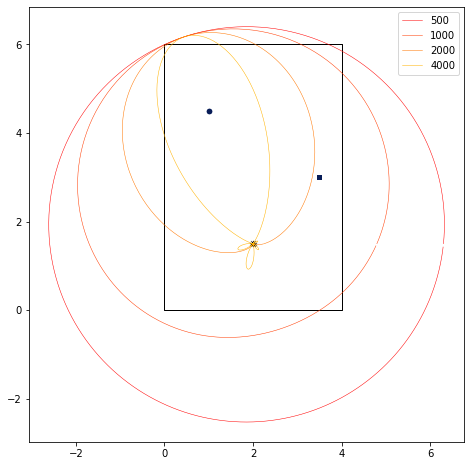Let's simulate the propagation and listen to the center microphone.

In :
room_bf.compute_rir()
room_bf.simulate()
print("Center Mic:")
IPython.display.Audio(room_bf.mic_array.signals[-1,:], rate=fs)

Center Mic:

Out:

Now let's see how simple DAS beamforming can improve the result.

In :
signal_das = mics.process(FD=False)
print("DAS Beamformed Signal:")
IPython.display.Audio(signal_das, rate=fs)

DAS Beamformed Signal:

Out: